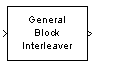# General Block Interleaver

Reorder symbols in input vector

## Library

Block sublibrary of Interleaving

•## Description

The General Block Interleaver block rearranges the elements of its input vector without repeating or omitting any elements. If the input contains N elements, then the Permutation vector parameter is a column vector of length N. The column vector indicates the indices, in order, of the input elements that form the length-N output vector; that is,

Output(k) = Input(Permutation vector(k))

for each integer k between 1 and N. The contents of Permutation vector must be integers between 1 and N, and must have no repetitions.

Both the input and the Permutation vector parameter must be column vector signals.

This block can output sequences that vary in length during simulation. For more information about sequences that vary in length, or variable-size signals, see Variable-Size Signal Basics (Simulink).

This block accept the following data types: `int8`, `uint8`, `int16`, `uint16`, `int32`, `uint32`, `boolean`, `single`, `double`, and fixed-point. The output signal inherits its data type from the input signal.

## Parameters

Permutation vector source

A selection that specifies the source of the permutation vector. The source can be either `Dialog` or ```Input port```. The default value is `Dialog`.

Permutation vector

A vector of length N that lists the indices of the output elements that came from the input vector. This parameter is available only when Permutation vector source is set to `Dialog`.

## Examples

If Permutation vector is `[4;1;3;2]` and the input vector is `[40;32;59;1]`, then the output vector is `[1;40;59;32]`. Notice that all of these vectors have the same length and that the vector Permutation vector is a permutation of the vector `[1:4]'`.

## Pair Block

General Block Deinterleaver

## Version History

Introduced before R2006a